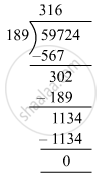# On dividing 59761 by a certain number, the quotient is 189 and the remainder is 37. Find the divisor. - Mathematics

Fill in the Blanks

On dividing 59761 by a certain number, the quotient is 189 and the remainder is 37. Find the divisor.

#### SolutionDividend = 59761, quotient = 189, remainder = 37 and divisor = ?
Dividend = divisor × quotient + remainder
⇒ 59761 = divisor × 189 + 37
⇒ 59761 − 37 = divisor × 189
⇒ 59724 = divisor × 189
⇒ Divisor = 59724 ​÷ 189
Hence, divisor =316

Concept: Concept for Whole Numbers
Is there an error in this question or solution?

#### APPEARS IN

RS Aggarwal Class 6 Mathematics
Chapter 3 Whole Numbers
Exercise 3E | Q 6 | Page 56